# Current Regulators: Construction, Working and Design Types

Published  June 5, 2019   0
E
Author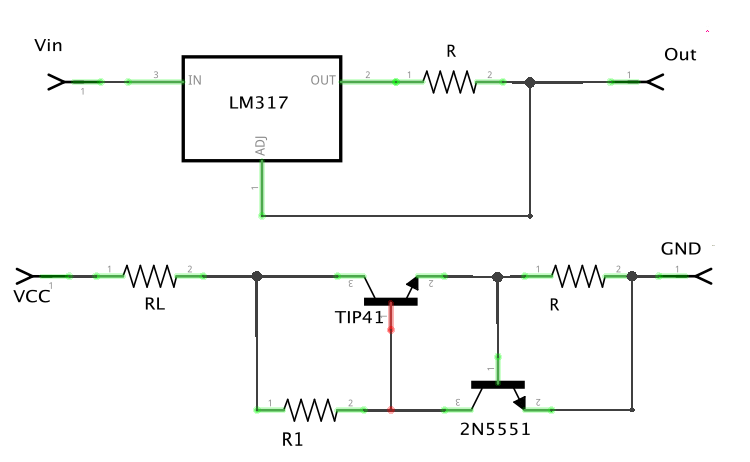Just like situations in which we need to regulate the voltage in our designs, there are scenarios where we need to regulate the current that’s being supplied to a particular part of our circuit. Unlike transforming (changing from one voltage level to another) which is usually one of the major reason for voltage regulation, current regulation is usually about keeping the current that’s being supplied constant, irrespective of variations in load resistance or input voltage. The circuits (integrated or not) which are used to achieve constant current supply are called (Constant) Current Regulators and they are very commonly used in Power Electronics.

While Current regulators have been featured in several applications over the years they are arguably not one of the most popular topics in electronics design conversations until recently. Current Regulators have now achieved a sort of ubiquitous status due to their important applications in LED Lighting among other applications.

For today's article, we will be looking at these current regulators and examine the operating principles behind them, their design, types, and applications among others.

### Operation Principle of Current Regulator

The operation of a current regulator is similar to that of the voltage regulator with the major difference being the parameter they regulate and the quantity they vary to supply their output. In voltage regulators, the current is varied to achieve the required voltage level, while current regulators usually involve variations in voltage/resistance to achieve the required current output. As such, while it is possible, it is usually difficult to regulate voltage and current at the same time in a circuit.

To understand how Current regulators work requires a quick look at ohms law;

`V=IR or I = V/R`

This means to maintain a constant current flow at an output, these two properties (voltage and resistance) must be kept constant in a circuit or adjusted such that when there is a change in one, the value of the other is adjusted accordingly to retain the same output current. As such, current regulation involves making an adjustment to either the voltage or resistance in a circuit or ensuring the Resistance and Voltage values are unchanged irrespective of the requirements/impacts of the connected load.

### Current Regulator Working

To properly describe how a current regulator operates, let’s consider the circuit diagram below.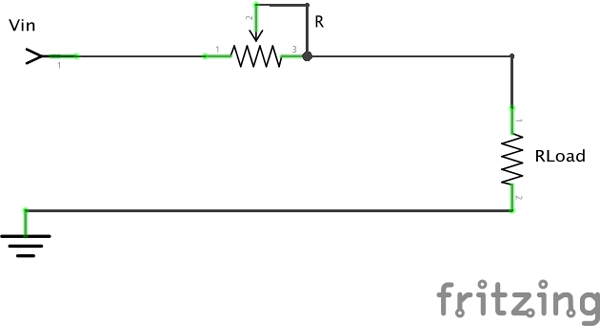The variable resistor in the circuit above is used to represent the actions of a current regulator. We will assume the variable resistor is automated and can auto-adjust its own resistance.    When the circuit is powered, the variable resistor adjusts its resistance to compensate for changes in the current due to variation in load resistance or voltage supply. From basic electricity class, you should remember that when the load, which is essentially resistance (+ capacitance/inductance) is increased, an effective drop in current is experienced and vice versa. Thus when the load in the circuit is increased (increase in resistance), rather than a current drop, the variable resistor reduces its own resistance to compensate for the increased resistance and ensure the same current flows. In the same way, when the load resistance reduces, the variable resistance increases its own resistance to compensate for the reduction, thus maintaining the output current value.

Another approach in current regulation is to connect a sufficiently high resistor in parallel with the load such that, in line with the laws of basic electricity, current will flow through the path with least resistance which in this case will be through the load, with only a "negligible" amount of current flowing through the high-value resistor.

These variations also affect the voltage as some current regulators maintain current at the output by varying the voltage. Thus, it’s almost impossible to regulate the voltage at the same output where the current is being regulated.

### Current Regulators Design

Current regulators are usually implemented using IC based voltage regulators like the MAX1818 and the LM317 or by the use of jellybean passive and active components like transistors and Zener diodes.

### Designing Current Regulators using Voltage Regulators

For the design of current regulators using IC-based voltage regulator, the technique usually involves setting up voltage regulators to have a constant load resistance and Linear voltage regulators are usually used because, the voltage between the output of linear regulators and their ground is usually tightly regulated, as such, a fixed resistor can be inserted between the terminals such that a fixed current flows to the load. A good example of a design based on this was published in one of the EDN publications by Budge Ing In 2016.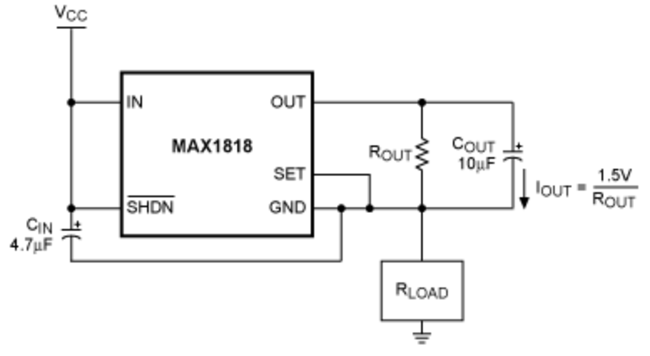The circuit employed uses the LDO linear regulator MAX1818 to create a high-side constant current regulated supply. The supply (shown in the image above) was designed such that it feeds RLOAD with a constant current, which is equal to   I = 1.5V/ROUT. Where 1.5V is the preset output voltage of the MAX1818 but can be changed using an external resistive divider.

To ensure the optimal performance of the design, the voltage at the input terminal of the MAX1818 must be up to 2.5V and not above 5.5v as this is the operating range stipulated by the datasheet. To satisfy that condition, choose a ROUT value that allows 2.5V to 5.5V between IN and GND. For example when a load of say 100Ω with a 5V VCC, the device functions properly with ROUT above 60Ω as the value allows a maximum programmable current of 1.5V/60Ω = 25mA. The voltage across the device then equals the minimum allowed: 5V - (25mA × 100Ω) = 2.5V.

Other linear regulators like the LM317 can also be used in a similar design process but one of the major benefits that ICs like the MAX1818 have over others is the fact that they incorporate thermal shutdown which could be very important in current regulation as the temperature of the IC tends to get hot when loads with high current requirements are connected.

For the LM317 based current regulator, consider the circuit below;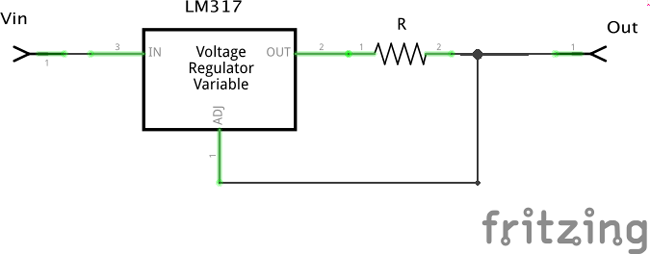LM317s are designed in such a way that the regulator keeps adjusting its voltage until the voltage between it's output pin and its adjustment pin is at 1.25v and as such a divider is usually used when implementing in a voltage regulator situation. But for our use case as a current regulator, it actually makes things super easy for us because, since the voltage is constant, all we need to do to make the current constant is to simply insert a resistor in series between the Vout and ADJ pin as shown in the circuit above. As such, we are able to set the output current to a fixed value which is given by;

```I = 1.25/R
```

With the value of R being the determinant factor of the output current value.

To create a variable current regulator, we only need to add a variable resistor to the circuit alongside another resistor to create a divider to the adjustable pin as shown in the image below.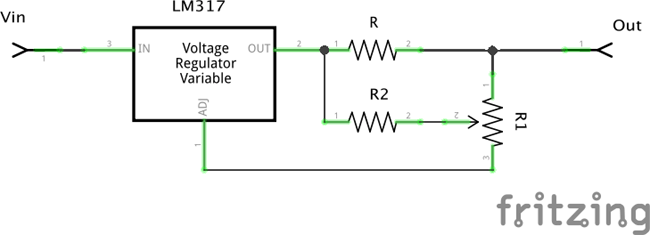The operation of the circuit is the same as the previous one with the difference being that the current can be adjusted in circuit by turning the knob of the potentiometer to vary the resistance. The voltage across R is giving by;

`V = (1 + R1/R2) x 1.25`

This means the Current across R is given by;

```IR = (1.25/R) x (1+ R1/R2).
```

This gives the circuit a current range of I = 1.25/R and (1.25/R) x (1 + R1/R2)

Depend on the set current; ensure the watt rating of resistor R can withstand the amount of current that will flow through it.

Below are some advantages for selecting the linear voltage regulator approach.

1. Regulator ICs incorporate over temperature protection which could come in handy when Loads with excessive current requirements are connected.
2. Regulator ICs have a greater tolerance for large input voltages and to a great extent support high power dissipation.
3. The regulator ICs approach involves the use of a smaller amount of components with the addition of only a few resistors in most cases except for cases where higher currents are required and power transistors are connected. This means you could use the same IC for voltage and current regulation.
4. The reduction in the number of components could mean a reduction in implementation cost and design time.

On the flip side, the configurations described under the regulator ICs approach allows the flow of quiescent current from the regulator to the load in addition to the regulated output voltage. This introduces an error which may not be permissible in certain applications. This could, however, be reduced by choosing a regulator with a very low quiescent current.

Another downside to the regulator IC approach is the lack of flexibility in the design.

Asides from the use of Voltage Regulator ICs, current regulators can also designed using jellybean parts including transistors, opamps, and Zener diode with necessary resistors. A Zener diode is used in the circuit probably as a no brainer as if you remember Zener diode is used for voltage regulation. Design of current regulator using these parts are the most flexible as they are usually easy to integrate into existing circuits.

### Current Regulator using Transistors

We will consider two designs under this section. The first one will feature the use of transistors only while the second will feature a mix of an operational amplifier and a power transistor.

For the one with Transistors, consider the circuit below.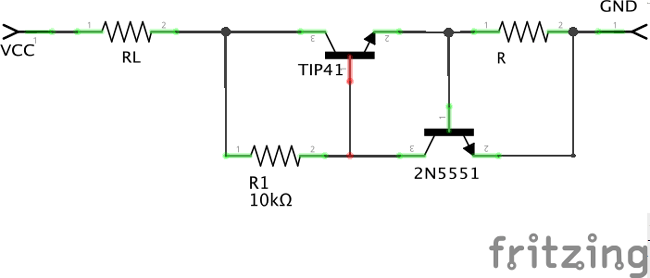The current regulator described in the circuit above is one of the simplest current regulator designs. It is a low-side current regulator; I connected after the load before the ground. It is made up of three key components; a control transistor (the 2N5551), a power transistor (The TIP41) and a shunt resistor(R). The shunt, which is essentially a low-value resistor, is used to measure the current flowing through the load. When the circuit is switched on, a voltage drop is noted across the shunt. The higher the value of the load resistance RL the higher the voltage drop across the shunt. The voltage drop across the shunt act as a trigger for the control transistor such that the higher the voltage drop across the shunt, the more the transistor conducts and regulates the bias voltage applied to the base of the power transistor to increase or reduce conduction with the resistor R1 acting as the bias resistor.

Just like with the other circuits, a variable resistor can be added in parallel to the shunt resistor to vary the current level by varying the amount of voltage applied at the base of the control transistor.

### Current Regulator using Op-Amp

For the second design path, consider the circuit below;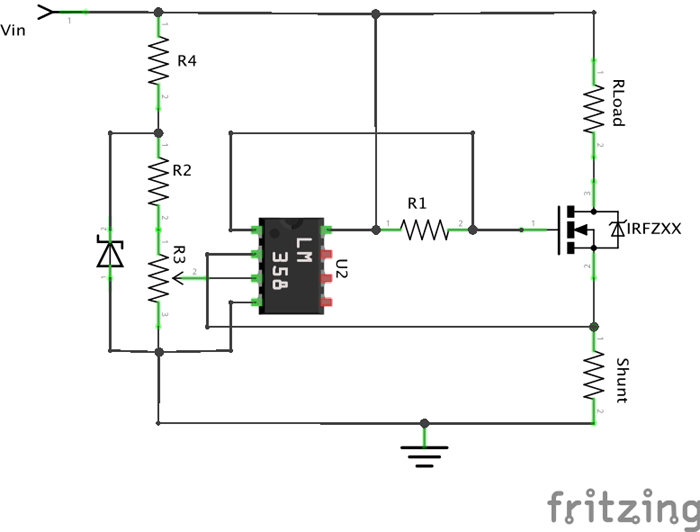This circuit is based on an operation amplifier, and just like in the example with the transistor, it also makes use of a shunt resistor for current sensing. The voltage drop across the shunt is fed into the operational amplifier which then compares it to a reference voltage set by the Zener diode ZD1. The op-amp compensates for any discrepancies (high or low) in the two input voltages by adjusting its output voltage. The output voltage of the operational amplifier is connected to a high power FET and conduction occurs based on the applied voltage.

The major difference between this design and the first one is the reference voltage implemented by the Zener diode. Both of these designs are linear and high amount of heat will be generated at high loads as such, heat sinks should be coupled to them to dissipate the heat.

The major advantage of this design approach is the flexibility it provides the designer. The parts can be selected and the design configured to taste without any of the limitations associated with internal circuitry which characterizes the regulator IC based approach.

On the other hand, this approach tends to be more tedious, time-consuming, requires more parts, bulky, susceptible to failing, and more expensive when compared with the regulator based IC approach.

### Application of Current Regulators

Constant current regulators find applications in all sorts of devices from power supply circuits, to Battery charging circuits, to LED drivers and other applications where a fixed current needs to be regulated irrespective of the applied Load.

That’s it for this article! Hope you learned one thing or two.

Till Next time!